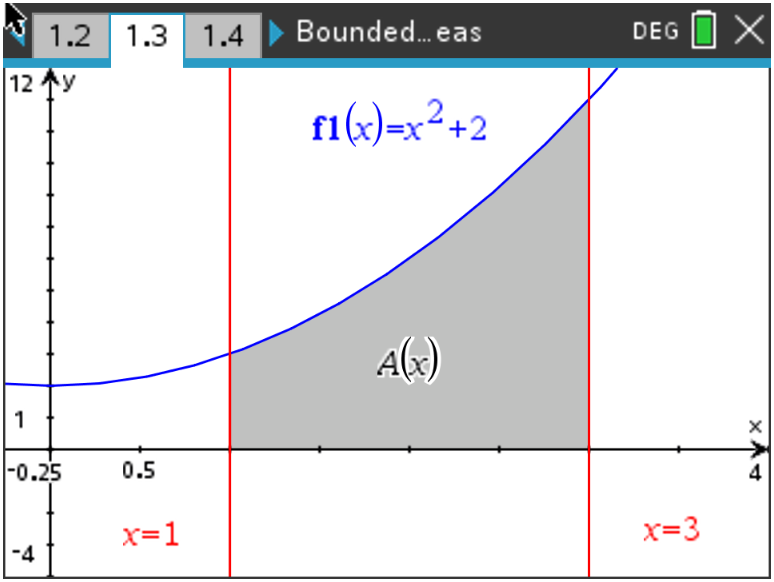# Activities

•• ##### Subject Area

• Math: Calculus: Definite Integrals and Applications

9-12

50 Minutes

• ##### Device
•TI-Nspire™ CX/CX II
•TI-Nspire™ CX CAS/CX II CAS
• ##### Software

TI-Nspire™ CX
TI-Nspire™ CX CAS

5.0

## Bounded Areas#### Activity Overview

In this lesson, students will compare the Riemann Sum area estimates with the exact bounded area of a curve and the x-axis.

#### Objectives

• Students will practice and discuss applications of finding the area bounded by a curve and the x-axis.
• Students will apply this information to finding the area bounded by two curves.
• Students will try to make a connection with how to understand these topics in both IB Mathematics and AP Calculus and on their final assessments.

#### Vocabulary

• Riemann Sums
• Underestimate
• Overestimate
• Definite Integrals
• Bounded Areas
• Concavity

#### About the Lesson

Students will use the subsequent pages of this TI-Nspire activity to refresh their skills on left-hand rectangle, right-hand rectangle, midpoint rectangle, and trapezoidal Riemann Sums given the bounded area between , the x-axis, and the vertical lines x = 1 and x = 3. For the remainder of this activity, we will call this bounded area .